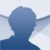Panecal Scientific Calculator Android

6.6.5

If you need a scientific calculator to carry out complex maths operations try downloading Panecal Scientific Calculator. It incorporates the typical advanced functions of this kind of calculator, as well as allowing you to work with different sorts of data and goals.

The tool for calculations in all sorts of tasks.

A very complete calculator

The main features of Panecal Scientific Calculator are the following and they make it a perfect work tool for calculations:

• Arithmetic, trigonometric, inverse trigonometric, exponential, logarithmic, power, power root and factorial functions.
• Editable and recallable expressions.
• Decimal, binary, octal and hexadecimal calculations.
• Primary memory and six memories for variables.
• Fixed and floating point.
• Engineering view modes.
• Configurable decimal separator.
• Landscape view mode.
• Key input confirmation by vibration.